Python package for concise, transparent, and accurate predictive modeling. All sklearn-compatible and easy to use.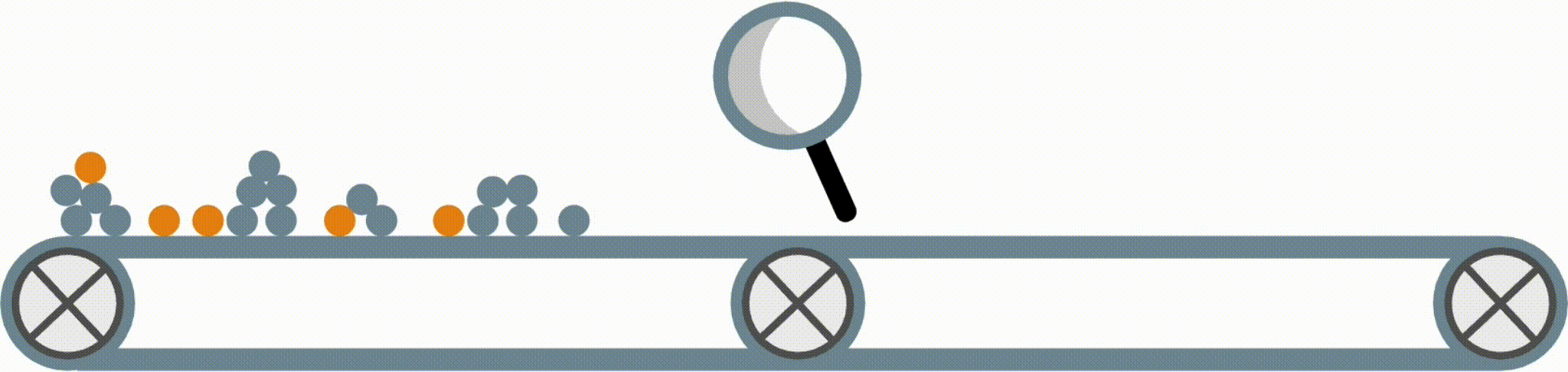Modern machine-learning models are increasingly complex, often making them difficult to interpret. This package provides a simple interface for fitting and using state-of-the-art interpretable models, all compatible with scikit-learn. These models can often replace black-box models (e.g. random forests) with simpler models (e.g. rule lists) while improving interpretability and computational efficiency, all without sacrificing predictive accuracy! Simply import a classifier or regressor and use the `fit` and `predict` methods, same as standard scikit-learn models.

``````from imodels import BoostedRulesClassifier, FIGSClassifier, SkopeRulesClassifier
from imodels import RuleFitRegressor, HSTreeRegressorCV, SLIMRegressor

model = BoostedRulesClassifier()  # initialize a model
model.fit(X_train, y_train)   # fit model
preds = model.predict(X_test) # predictions: shape is (n_test, 1)
preds_proba = model.predict_proba(X_test) # predicted probabilities: shape is (n_test, n_classes)
print(model) # print the rule-based model

-----------------------------
# the model consists of the following 3 rules
# if X1 > 5: then 80.5% risk
# else if X2 > 5: then 40% risk
# else: 10% risk
``````

### Installation

Install with `pip install imodels` (see here for help).

### Supported models

Model Reference Description
Rulefit rule set 🗂️, 🔗, 📄 Fits a sparse linear model on rules extracted from decision trees
Skope rule set 🗂️, 🔗 Extracts rules from gradient-boosted trees, deduplicates them,
then linearly combines them based on their OOB precision
Boosted rule set 🗂️, 🔗, 📄 Sequentially fits a set of rules with Adaboost
Slipper rule set 🗂️, ㅤㅤ📄 Sequentially learns a set of rules with SLIPPER
Bayesian rule set 🗂️, 🔗, 📄 Finds concise rule set with Bayesian sampling (slow)
Optimal rule list 🗂️, 🔗, 📄 Fits rule list using global optimization for sparsity (CORELS)
Bayesian rule list 🗂️, 🔗, 📄 Fits compact rule list distribution with Bayesian sampling (slow)
Greedy rule list 🗂️, 🔗 Uses CART to fit a list (only a single path), rather than a tree
OneR rule list 🗂️, ㅤㅤ📄 Fits rule list restricted to only one feature
Optimal rule tree 🗂️, 🔗, 📄 Fits succinct tree using global optimization for sparsity (GOSDT)
Greedy rule tree 🗂️, 🔗, 📄 Greedily fits tree using CART
C4.5 rule tree 🗂️, 🔗, 📄 Greedily fits tree using C4.5
TAO rule tree 🗂️, ㅤㅤ📄 Fits tree using alternating optimization
Iterative random
forest
🗂️, 🔗, 📄 Repeatedly fit random forest, giving features with
high importance a higher chance of being selected
Sparse integer
linear model
🗂️, ㅤㅤ📄 Sparse linear model with integer coefficients
Greedy tree sums 🗂️, ㅤㅤ📄 Sum of small trees with very few total rules (FIGS)
Hierarchical
shrinkage wrapper
🗂️, ㅤㅤ📄 Improve any tree-based model with ultra-fast, post-hoc regularization
Distillation
wrapper
🗂️ Train a black-box model,
then distill it into an interpretable model
More models (Coming soon!) Lightweight Rule Induction, MLRules, …

Docs 🗂️, Reference code implementation 🔗, Research paper 📄

## What's the difference between the models?

The final form of the above models takes one of the following forms, which aim to be simultaneously simple to understand and highly predictive:

Rule set Rule list Rule tree Algebraic models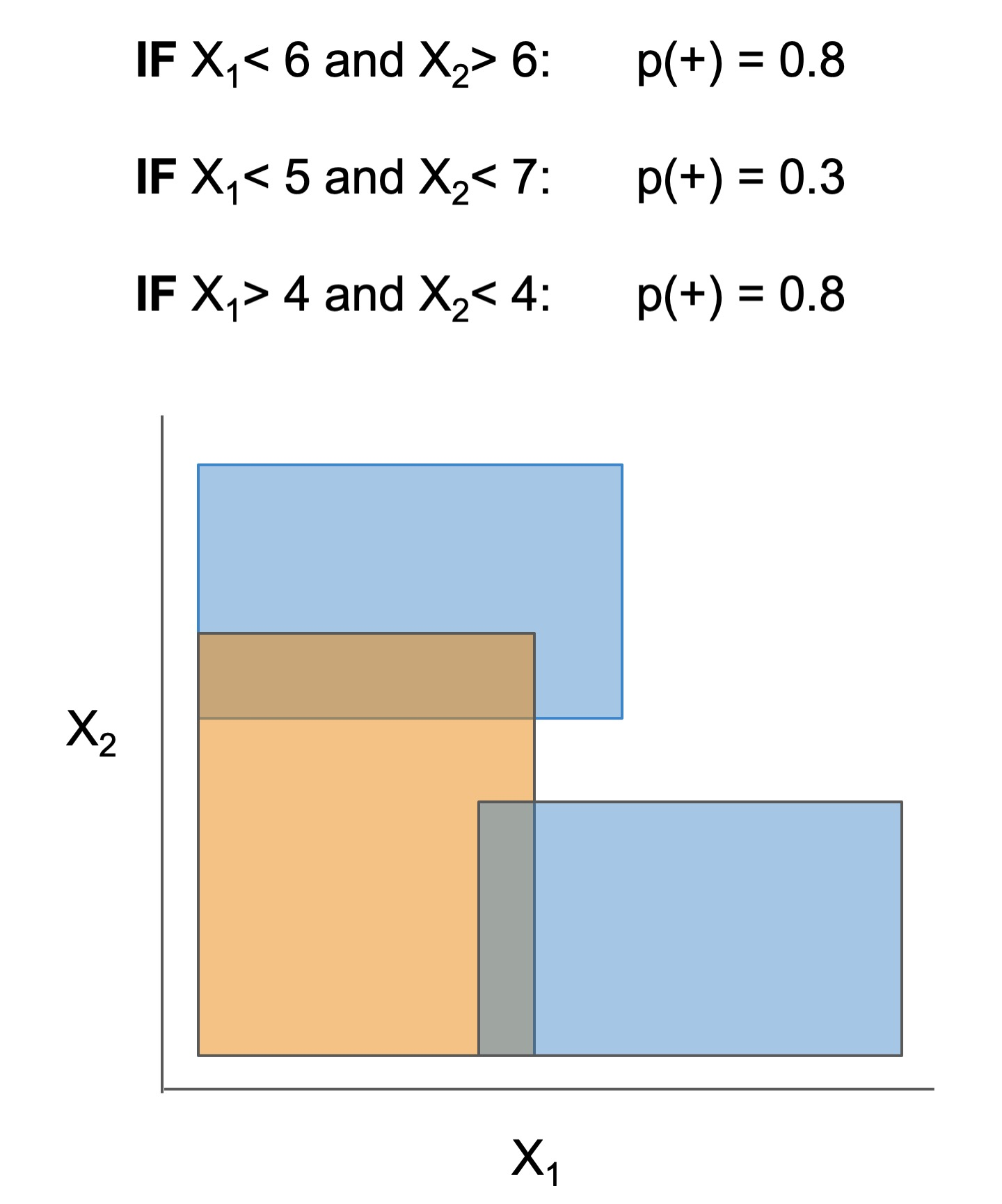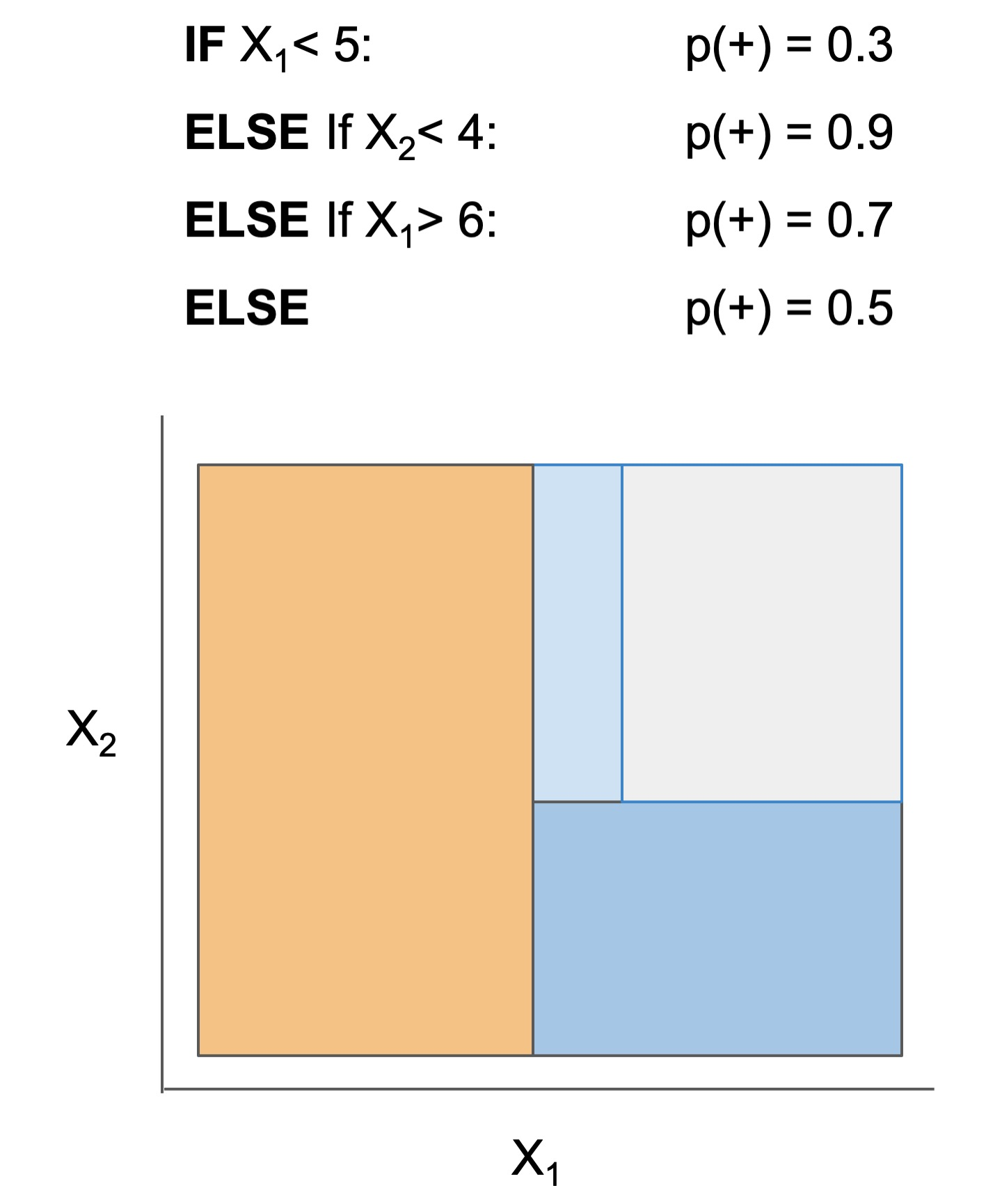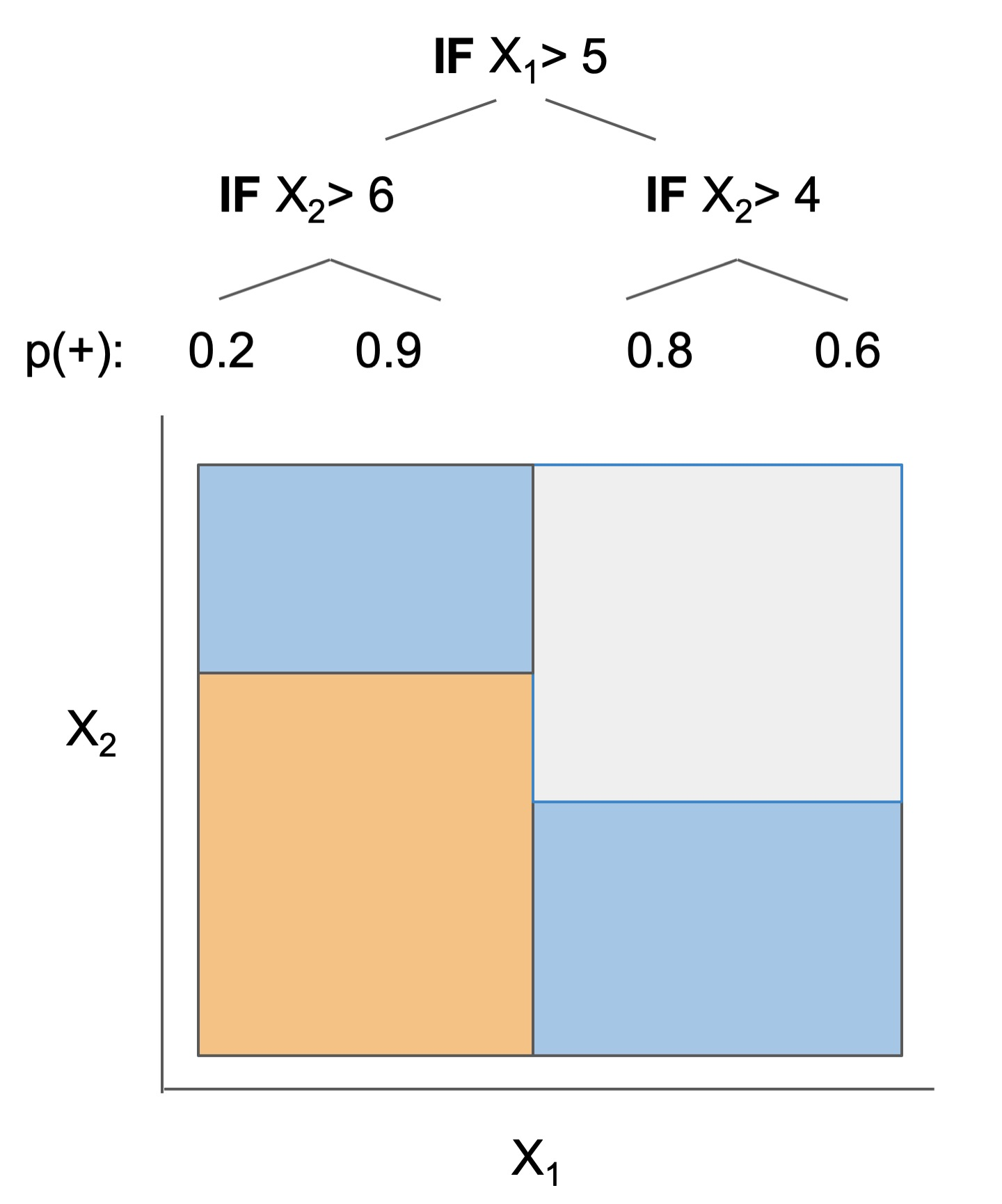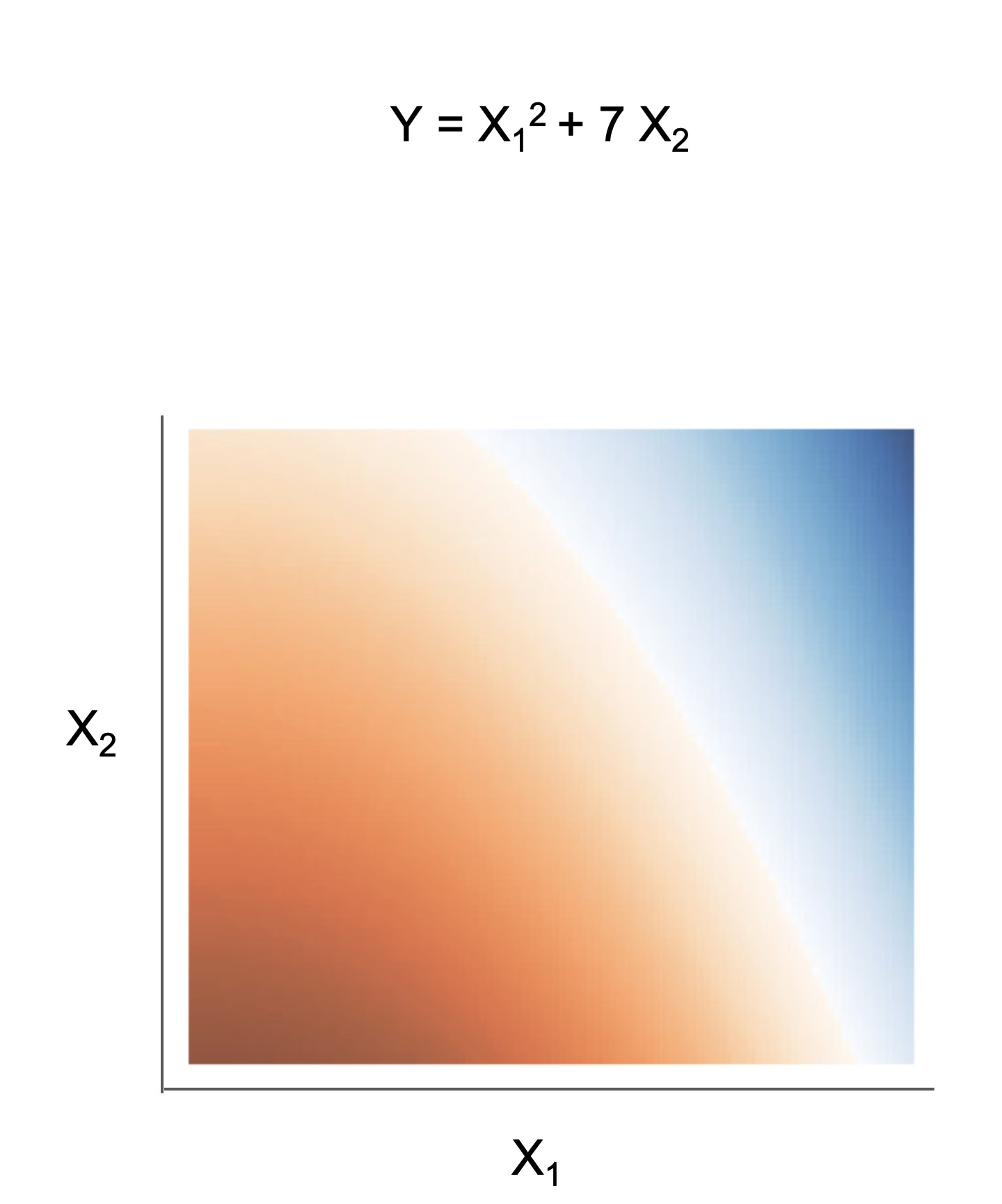Different models and algorithms vary not only in their final form but also in different choices made during modeling, such as how they generate, select, and postprocess rules:

Rule candidate generation Rule selection Rule postprocessing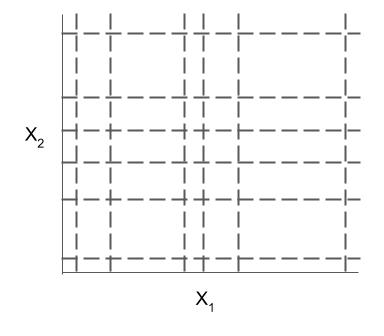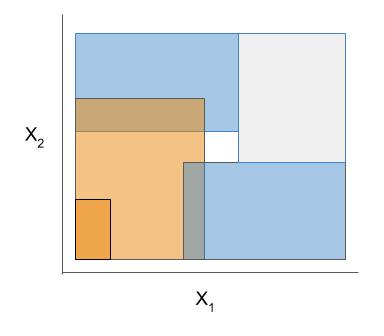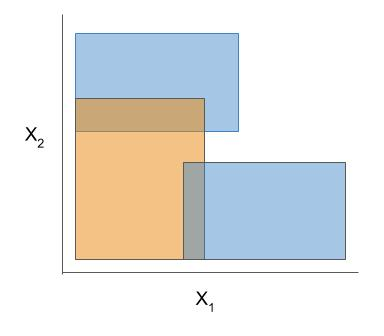Ex. RuleFit vs. SkopeRules RuleFit and SkopeRules differ only in the way they prune rules: RuleFit uses a linear model whereas SkopeRules heuristically deduplicates rules sharing overlap.
Ex. Bayesian rule lists vs. greedy rule lists Bayesian rule lists and greedy rule lists differ in how they select rules; bayesian rule lists perform a global optimization over possible rule lists while Greedy rule lists pick splits sequentially to maximize a given criterion.
Ex. FPSkope vs. SkopeRules FPSkope and SkopeRules differ only in the way they generate candidate rules: FPSkope uses FPgrowth whereas SkopeRules extracts rules from decision trees.

## Demo notebooks

Demos are contained in the notebooks folder.

Quickstart demo Shows how to fit, predict, and visualize with different interpretable models
Autogluon demo Fit/select an interpretable model automatically using Autogluon AutoML
Quickstart colab demoShows how to fit, predict, and visualize with different interpretable models
Clinical decision rule notebook Shows an example of using `imodels` for deriving a clinical decision rule
Posthoc analysis We also include some demos of posthoc analysis, which occurs after fitting models: posthoc.ipynb shows different simple analyses to interpret a trained model and uncertainty.ipynb contains basic code to get uncertainty estimates for a model

Different models support different machine-learning tasks. Current support for different models is given below (each of these models can be imported directly from imodels (e.g. `from imodels import RuleFitClassifier`):

Model Binary classification Regression Notes
Rulefit rule set RuleFitClassifier RuleFitRegressor
Skope rule set SkopeRulesClassifier
Boosted rule set BoostedRulesClassifier
SLIPPER rule set SlipperClassifier
Bayesian rule set BayesianRuleSetClassifier Fails for large problems
Optimal rule list (CORELS) OptimalRuleListClassifier Requires corels, fails for large problems
Bayesian rule list BayesianRuleListClassifier
Greedy rule list GreedyRuleListClassifier
OneR rule list OneRClassifier
Optimal rule tree (GOSDT) OptimalTreeClassifier Requires gosdt, fails for large problems
Greedy rule tree (CART) GreedyTreeClassifier GreedyTreeRegressor
C4.5 rule tree C45TreeClassifier
TAO rule tree TaoTreeClassifier TaoTreeRegressor
Iterative random forest IRFClassifier Requires irf
Sparse integer linear model SLIMClassifier SLIMRegressor Requires extra dependencies for speed
Greedy tree sums (FIGS) FIGSClassifier FIGSRegressor
Hierarchical shrinkage HSTreeClassifierCV HSTreeRegressorCV Wraps any sklearn tree-based model
Distillation DistilledRegressor Wraps any sklearn-compatible models

### Extras

Data-wrangling functions for working with popular tabular datasets (e.g. compas). These functions, in conjunction with imodels-data and imodels-experiments, make it simple to download data and run experiments on new models.
Explain classification errors with a simple posthoc function. Fit an interpretable model to explain a previous model's errors (ex. in this notebook📓).
Fast and effective discretizers for data preprocessing.
Discretizer Reference Description
MDLP 🗂️, 🔗, 📄 Discretize using entropy minimization heuristic
Simple 🗂️, 🔗 Simple KBins discretization
Random Forest 🗂️ Discretize into bins based on random forest split popularity
Rule-based utils for customizing models The code here contains many useful and customizable functions for rule-based learning in the util folder. This includes functions / classes for rule deduplication, rule screening, and converting between trees, rulesets, and neural networks.

## Our favorite models

After developing and playing with `imodels`, we developed a few new models to overcome limitations of existing interpretable models.

### FIGS: Fast interpretable greedy-tree sums

Fast Interpretable Greedy-Tree Sums (FIGS) is an algorithm for fitting concise rule-based models. Specifically, FIGS generalizes CART to simultaneously grow a flexible number of trees in a summation. The total number of splits across all the trees can be restricted by a pre-specified threshold, keeping the model interpretable. Experiments across a wide array of real-world datasets show that FIGS achieves state-of-the-art prediction performance when restricted to just a few splits (e.g. less than 20).Example FIGS model. FIGS learns a sum of trees with a flexible number of trees; to make its prediction, it sums the result from each tree.

### Hierarchical shrinkage: post-hoc regularization for tree-based methods

Hierarchical shrinkage is an extremely fast post-hoc regularization method which works on any decision tree (or tree-based ensemble, such as Random Forest). It does not modify the tree structure, and instead regularizes the tree by shrinking the prediction over each node towards the sample means of its ancestors (using a single regularization parameter). Experiments over a wide variety of datasets show that hierarchical shrinkage substantially increases the predictive performance of individual decision trees and decision-tree ensembles.

• Interpretable ML good quick overview: murdoch et al. 2019, pdf
• Interpretable ML book: molnar 2019, pdf
• Case for interpretable models rather than post-hoc explanation: rudin 2019, pdf
• Review on evaluating interpretability: doshi-velez & kim 2017, pdf
Reference implementations (also linked above) The code here heavily derives from the wonderful work of previous projects. We seek to to extract out, unify, and maintain key parts of these projects.
Related packages
• gplearn: symbolic regression/classification
• pysr: fast symbolic regression
• interpretml: boosting-based gam
• h20 ai: gams + glms (and more)
• optbinning: data discretization / scoring models
• For updates, star the repo, see this related repo, or follow @csinva_
• Please make sure to give authors of original methods / base implementations appropriate credit!
• Contributing: pull requests very welcome!

If it's useful for you, please star/cite the package, and make sure to give authors of original methods / base implementations credit:

``````@software{
imodels2021,
title        = {imodels: a python package for fitting interpretable models},
journal      = {Journal of Open Source Software},
publisher    = {The Open Journal},
year         = {2021},
author       = {Singh, Chandan and Nasseri, Keyan and Tan, Yan Shuo and Tang, Tiffany and Yu, Bin},
volume       = {6},
number       = {61},
pages        = {3192},
doi          = {10.21105/joss.03192},
url          = {https://doi.org/10.21105/joss.03192},
}

``````
Expand source code
``````"""
"""
# Python `imodels` package for interpretable models compatible with scikit-learn.
# Github repo available [here](https://github.com/csinva/imodels)

from .algebraic.slim import SLIMRegressor, SLIMClassifier
from .discretization.discretizer import RFDiscretizer, BasicDiscretizer
from .discretization.mdlp import MDLPDiscretizer, BRLDiscretizer
from .experimental.bartpy import BART
from .rule_list.bayesian_rule_list.bayesian_rule_list import BayesianRuleListClassifier
from .rule_list.corels_wrapper import OptimalRuleListClassifier
from .rule_list.greedy_rule_list import GreedyRuleListClassifier
from .rule_list.one_r import OneRClassifier
from .rule_set import boosted_rules
from .rule_set.boosted_rules import *
from .rule_set.boosted_rules import BoostedRulesClassifier
from .rule_set.brs import BayesianRuleSetClassifier
from .rule_set.fplasso import FPLassoRegressor, FPLassoClassifier
from .rule_set.fpskope import FPSkopeClassifier
from .rule_set.rule_fit import RuleFitRegressor, RuleFitClassifier
from .rule_set.skope_rules import SkopeRulesClassifier
from .rule_set.slipper import SlipperClassifier
from .tree.c45_tree.c45_tree import C45TreeClassifier
from .tree.cart_ccp import DecisionTreeCCPClassifier, DecisionTreeCCPRegressor, HSDecisionTreeCCPClassifierCV, \
HSDecisionTreeCCPRegressorCV
# from .tree.iterative_random_forest.iterative_random_forest import IRFClassifier
# from .tree.optimal_classification_tree import OptimalTreeModel
from .tree.cart_wrapper import GreedyTreeClassifier, GreedyTreeRegressor
from .tree.figs import FIGSRegressor, FIGSClassifier, FIGSRegressorCV, FIGSClassifierCV
from .tree.gosdt.pygosdt import OptimalTreeClassifier
from .tree.gosdt.pygosdt_shrinkage import HSOptimalTreeClassifier, HSOptimalTreeClassifierCV
from .tree.hierarchical_shrinkage import HSTreeRegressor, HSTreeClassifier, HSTreeRegressorCV, HSTreeClassifierCV
from .tree.tao import TaoTreeClassifier, TaoTreeRegressor
from .util.data_util import get_clean_dataset
from .util.distillation import DistilledRegressor
from .util.explain_errors import explain_classification_errors
from .importance.representation import TreeTransformer

CLASSIFIERS = [BayesianRuleListClassifier, GreedyRuleListClassifier, SkopeRulesClassifier,
BoostedRulesClassifier, SLIMClassifier, SlipperClassifier, BayesianRuleSetClassifier,
C45TreeClassifier, OptimalTreeClassifier, OptimalRuleListClassifier, OneRClassifier,
SlipperClassifier, RuleFitClassifier, TaoTreeClassifier,
FIGSClassifier, HSTreeClassifier, HSTreeClassifierCV]  # , IRFClassifier
REGRESSORS = [RuleFitRegressor, SLIMRegressor, GreedyTreeClassifier, FIGSRegressor,
TaoTreeRegressor, HSTreeRegressor, HSTreeRegressorCV, BART]
DISCRETIZERS = [RFDiscretizer, BasicDiscretizer, MDLPDiscretizer, BRLDiscretizer]``````